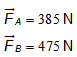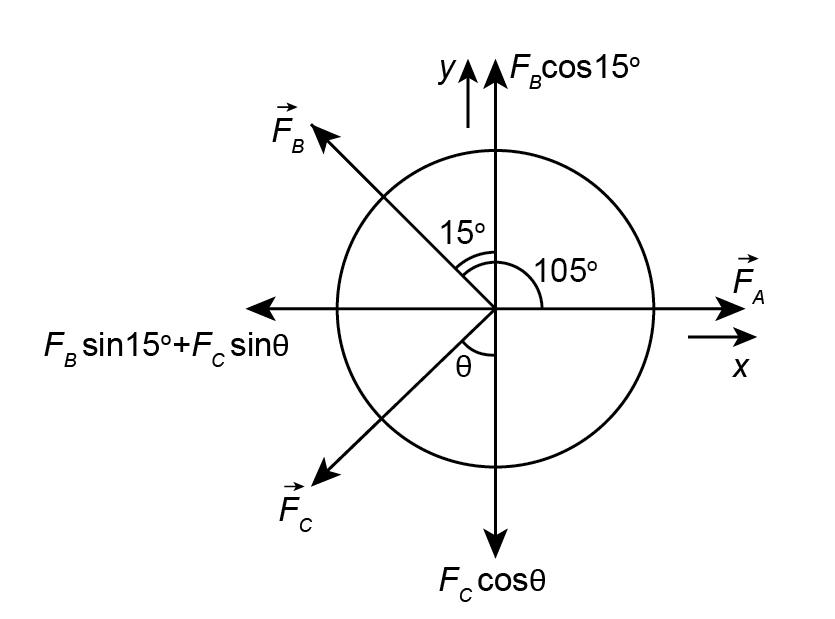×
Get Full Access to Physics: Principles With Applications - 7 Edition - Chapter 9 - Problem 9.1
Get Full Access to Physics: Principles With Applications - 7 Edition - Chapter 9 - Problem 9.1

×

# Three forces are applied to a tree sapling, as | Ch 9 - 9.1ISBN: 9780321625922 81

## Solution for problem 9.1 Chapter 9

Physics: Principles with Applications | 7th Edition

• Textbook Solutions
• 2901 Step-by-step solutions solved by professors and subject experts
• Get 24/7 help from StudySoup virtual teaching assistantsPhysics: Principles with Applications | 7th Edition

4 5 1 240 Reviews
13
2
Problem 9.1

Three forces are applied to a tree sapling, as shown in Fig. 946, to stabilize it. If and find in magnitude and direction.

Step-by-Step Solution:

Step 1 of 4

Given data:.

Force diagram:Step 2 of 4

Step 3 of 4

##### ISBN: 9780321625922

This full solution covers the following key subjects: . This expansive textbook survival guide covers 33 chapters, and 9632 solutions. This textbook survival guide was created for the textbook: Physics: Principles with Applications, edition: 7. The answer to “Three forces are applied to a tree sapling, as shown in Fig. 946, to stabilize it. If and find in magnitude and direction.” is broken down into a number of easy to follow steps, and 23 words. Since the solution to 9.1 from 9 chapter was answered, more than 292 students have viewed the full step-by-step answer. Physics: Principles with Applications was written by and is associated to the ISBN: 9780321625922. The full step-by-step solution to problem: 9.1 from chapter: 9 was answered by , our top Physics solution expert on 09/04/17, 10:13PM.

## Discover and learn what students are asking

Statistics: Informed Decisions Using Data : Discrete Random Variables
?What are the two requirements for a discrete probability distribution?

Statistics: Informed Decisions Using Data : Discrete Probability Distributions
?Determine whether the distribution is a discrete probability distribution. If not, state why.

Statistics: Informed Decisions Using Data : An Overview of Nonparametric Statistics
?Describe the difference between parametric statistical procedures and nonparametric statistical procedures.

Unlock Textbook Solution